# Choosing a Motor and Gearing Combination

Wednesday - 20/12/2017 09:39
For most robotics and mechatronics applications, DC motors run at speeds higher than we want and with less torque than we want. The most common solution to this problem is to attach the output shaft of the motor to a transmission that reduces speed and increases torque.

The input to this transmission is attached to the output shaft of the motor, and the output of the transmission performs the lower speed, higher torque motion that we want (e.g., motion of a wheel, robot joint, etc.). The transmission can be built of belts and pulleys, gears, or other elements, and the output motion of the transmission can be rotational or linear.

Here, let's consider the case of a rotational output motion where the output speed of the transmission is G times slower than the input speed of the transmission (the speed of the motor shaft). Since gearboxes are the most common transmission, we call G the gear ratio, and usually G > 1. We will refer to transmissions as gearboxes.

If the input shaft to an ideal gearbox is turning with a speed ωin and torque τin, then the output turns with a speed ωout and torque τout, where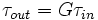$\tau_{out} = G \tau_{in}\,$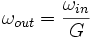$\omega_{out} = \frac{\omega_{in}}{G}$

We see that the output power Pout equals the input power Pin: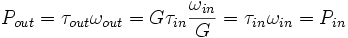$''P_{out} = \tau_{out} \omega_{out} = G \tau_{in} \frac{\omega_{in}}{G} = \tau_{in} \omega_{in} = P_{in}''$

since the ideal gearbox neither adds nor subtracts power. If the speed ω is measured in radians per second, and the torque τ is measured in Newton-meters, then the units for power are Watts.

In terms of the speed-torque curve above, a gearbox has the effect of reducing the maximum speed and increasing the maximum torque. The figure below shows a speed-torque curve of a motor, and for the same motor with gearboxes of G = 2 and G = 4. Also shown is a desired speed+torque operating point for the motor+gearbox combination, showing that only the G = 4 gearbox meets our specification.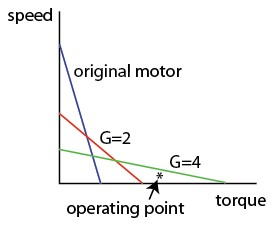If we have two gearboxes with gear ratios G1 and G2, and the output shaft of gearbox 1 is connected to the input shaft of gearbox 2, the total gear ratio from the input of gearbox 1 to the output of gearbox 2 is Gtotal = G1G2. Gearboxes can achieve gear ratios of several hundred, often by chaining multiple sets of gears.

In practice, transmissions are not 100% efficient at transmitting power. Some power is lost due to friction. Gearboxes have an associated efficiency η < 1, such that Pout = ηPin. Since the gear exactly enforces the speed relationship ωout = ωin / G, this means that the torque output of the gearbox is actually τout = ηGτin.

The efficiencies of gearboxes attached to each other is obtained by multiplying the individual efficiencies. Gearboxes with large gear ratios are usually obtained by having several gearing stages in series, so the total efficiency of large gear ratio gearboxes are generally lower than those with smaller gear ratios (if the gears are made with the same materials and precision). Below is shown the speed-torque curve for a motor, a motor with an ideal gearbox with G = 2, and a motor with a gearbox with G = 2 but an efficiency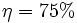$\eta = 75\%$.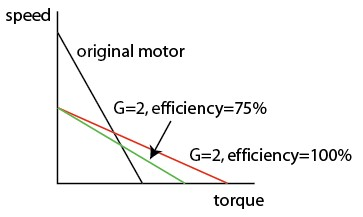Another practical issue with gearboxes is backlash. Backlash is defined as the angle the gearbox output shaft can be rotated without the input shaft moving. Backlash can be large (a few degrees) in cheap gears, much lower in precision gears, and nearly zero for certain gear technologies (e.g., harmonic drive gears). See the Gears page.

One other issue to keep in mind with gearboxes is backdrivability. A motor+gearbox is backdrivable if it is possible to rotate the output of the gearbox while the motor is off. Some gear types make it nearly impossible to backdrive. Sometimes you want the system to be backdrivable, so a human can physically manipulate it, for example.

Other times you don't: for example, you might want the system to hold its position without brakes or power, even under gravity. Backdrivability is a qualitative property. Of two systems using the same type of gearing, the one with the smaller gear ratio is usually more backdrivable.

Finally, gearboxes usually come with specifications on the maximum speed allowable at the input shaft and the maximum torque allowable at the output shaft.

To choose a motor and gearbox appropriate for your application, consider the following issues:

• What is the typical power you need? In other words, what is a typical speed and torque operating point for your system? Choose a motor that is capable of providing this much mechanical power. (Most motors are classified according to their power.) Usually you will choose the smallest, lightest, most inexpensive motor that meets your specifications. You may also have constraints on the nominal voltage of the motor (e.g., you have a 12V supply available, so you want the motor to run at around 12V). Since the input electrical power is the current times the voltage, low-voltage-rated motors of the same power draw larger amounts of current.

• Once you have chosen a motor, choose a gearbox for the motor so that the speed+torque combinations you want from it (including the maximum speed and maximum torque you need) are under the speed-torque curve of the motor+gearbox combination. Also make sure the gearbox output shaft maximum torque specification is sufficient.
• Motors should not be run for long periods of time at stall, as they are likely to overheat, as explained in Brushed DC Motor Theory. It is fine if they intermittently stall. The allowable continuous operation region of the speed-torque curve depends on the thermal characteristics of your motor, but typically you don't want continuous operation at less than 1/4 or 1/2 of the maximum speed of the motor. You may need to choose a larger motor to meet this specification.
• You can further optimize your design for maximum efficiency in converting electrical power to mechanical power, to save on electrical power for battery-powered robots. Motors usually are most efficient at converting electrical power to mechanical power at high speeds. On the other hand, gearboxes with larger gear ratios generally have lower efficiency than gearboxes with smaller gear ratios. Don't worry about efficiency in converting electrical to mechanical power unless it is a critical issue for you.
• Some of the mechanical power created by a motor goes to accelerate the rotor of the motor itself, and some of it goes to accelerate the load. To maximize the mechanical power that goes to accelerate the load, you would choose a gear ratio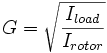$G = \sqrt{\frac{I_{load}}{I_{rotor}}}$

This is called inertia matching. Again, this is only an issue for more sophisticated designs.• Support

Sales Manager

+(84)923.886.133

dolin@dolin.com.vn

Sales 01

+(84)918.26.11.44

dolinvn@dolin.com.vn

Sales 02

+(84)941.976.189

info@dolin.com.vn

Sales 03

+(84)987.246.757

sales@dolin.com.vn

Sales 04

+(84)949.380.913

dolinsales@dolin.com.vn

Sales 05

+(84)934.008.489

dolinvn@dolin.vn

Bestsellers
Construction and Land
Counter
• Online29
• Today497
• This month222,488
• Total22,195,213You did not use the site, Click here to remain logged. Timeout: 60 second